Ch 9. 3-D Motion of a Rigid Body Multimedia Engineering Dynamics Translating Coordinates Rotating Coordinates Inertia & Ang. Momentum Equationsof Motion
 Chapter - Particle - 1. General Motion 2. Force & Accel. 3. Energy 4. Momentum - Rigid Body - 5. General Motion 6. Force & Accel. 7. Energy 8. Momentum 9. 3-D Motion 10. Vibrations Appendix Basic Math Units Basic Equations Sections Search eBooks Dynamics Fluids Math Mechanics Statics Thermodynamics Author(s): Kurt Gramoll ©Kurt GramollDYNAMICS - CASE STUDY SOLUTION Solution of a) Begin with a diagram, modeling the hull as a circular cylinder and the con as a rectangular prism. Use Eqs. 1 to determine the moments of inertia of the con about its center of gravity: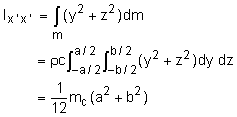(Note, dm = ρ c dy dz) Using a similar derivation, we get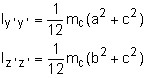These expressions for the moment of inertia could also be found from Appendix B, or a table of inertial properties. Equations 2 give the products of inertia of the con about its center of gravity: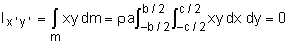(Note, dm = ρ a dx dy) Using a similar derivation, we get      Iy'z' = Ix'z' = 0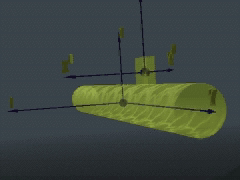Problem Simplification- Skew View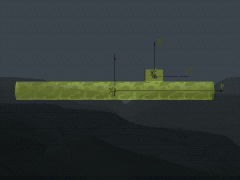Problem Simplification - Side View The expressions for the products of inertia could have been determined simply by noting that the product of inertia about a plane of symmetry is always zero. Use the parallel axis theorem (Eqs. 3) to transfer the moment of inertia of the con about its center of gravity to the center of gravity of the sub:      Ixx = Ix'x' + mc (dy2 + dz2)           = 1/12 mc(a2 + b2) + mc (dy2 + dz2)      Iyy = 1/12 mc(a2 + c2) + mc (dx2 + dz2)      Izz = 1/12 mc(b2 + c2) + mc (dx2 + dy2) Use the parallel plane theorem (Eqs. 4) to transfer the product of inertia of the con about its center of gravity to the center of gravity of the sub:      Ixy = Ix'y' + mrdxdy      Iyz = mcdydz      Ixz = 0 Solution of b) Use Eq. 8 to express the angular momentum of the con about its center of gravity (Eq. 5) in scalar form:      Hcg = Hxi + Hyj + Hzk Here, we have      Hx = -Ixxωx      Hy = Iyyωy      Hz = -Iyzωy Express the position and velocity of the con in rectangular components, and determine the moment of the linear momentum of the con about the center of gravity of the sub:      ρcon = dyj + dzk      vcon = vxj + vyk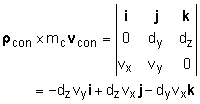Use Eq. 7 to determine the angular momentum of the con about the center of gravity of the sub:      HA = ρcon × mcvcon + Hcg             = (-Ixxωx - dzvy)i + (-Iyyωy - dzvx)j                      + (-Iyzωy - dyvx)k

Practice Homework and Test problems now available in the 'Eng Dynamics' mobile app
Includes over 400 problems with complete detailed solutions.
Available now at the Google Play Store and Apple App Store.